Prev Up Next

# Colouring

Forcing chains, discussed earlier, and to be discussed in more detail and greater generality later, give a concise way of showing why a particular cell cannot have a particular value.

But while solving, one does not proceed in a linear fashion. The linear chain is an extract of the non-linear work done before. Colouring is a way to add mark-up to the grid (explicitly or not) in order to facilitate finding forcing chains.

## Simple colouring

The simplest version goes like this: Pick a digit d, and make a graph on the cells with that digit as candidate, joining two when they are adjacent (i.e., occur in the same row, column or box, so that at most one is d) and moreover form a pair for d (so that at least one is d). Now for every edge in the graph precisely one end is d. A colour is a connected component of this graph.

The graph is bipartite, and the set of digits d in the solution forms one part of a bipartition of the graph. If a cell is adjacent to two cells in the same connected component but in different parts of the bipartition, then that cell does not have the value d.

Note that simple colouring is a single digit technique. If you are good at Nishio, simple colouring is superfluous, Nishio will do it all for you. On the other hand, simple colouring includes techniques such as Turbot Fish.

## Example

This example was given by Lummox JR. By the usual arguments it is completed to the second diagram.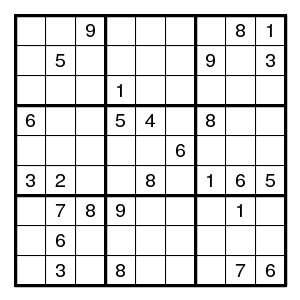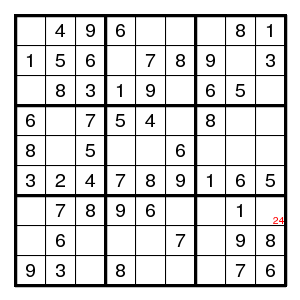Now look at the possibilities for a digit 4. Color the cells in one component two shades of yellow, in another component two shades of green, etc. - one shade in one part of the bipartition, one in the other. The grey cells cannot have a 4. Six cells are not coloured because they form components of size 1.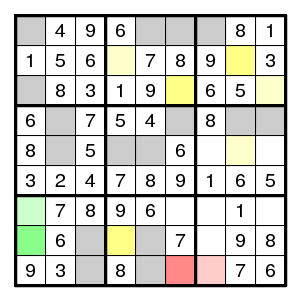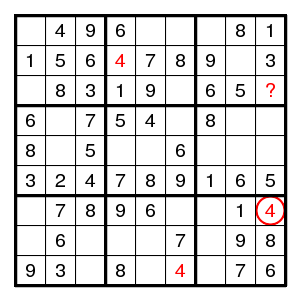We know that for each colour, one of the two shades represents the true solution. Now row 8 shows that darker yellow and darker green are not both true. So either lighter yellow or lighter green is true. But in both cases cell (7,9) is false, i.e., (7,9)!4. This follows also by looking at column 6: darker yellow and darker red are not both true, but (7,9) sees both lighter yellow and lighter red, so again (7,9)!4.

From the colouring point of view both arguments are equally easy. But if we extract the corresponding forcing chains, then the first one reads (7,9)-(7,1)=(8,1)-(8,4)=(2,4)-(2,8)=(3,9)-(7,9) and the second one is the much shorter Turbot Fish (7,9)-(9,7)=(9,6)-(3,6)=(3,9)-(7,9).

We also give the Nishio point-of-view: (7,9)4 forces (9,6)4 and (2,4)4 and there is no room for a 4 in the upper right hand corner.

That was one exclusion. Now (7,9)2 and we progress easily until the next non-trivial stage. This time it is a good idea to colour possible occurrences of the digit 2.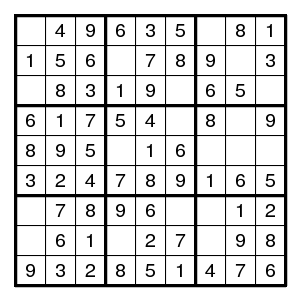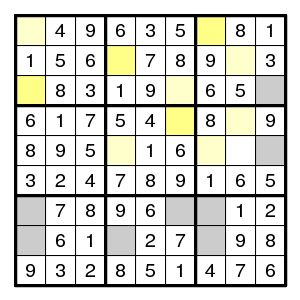Here two lighter yellow cells see each other, so lighter yellow is false and darker yellow is true. Now all is straightforward.

## Swordfish

The following position was given by simes. Here first a direct solution.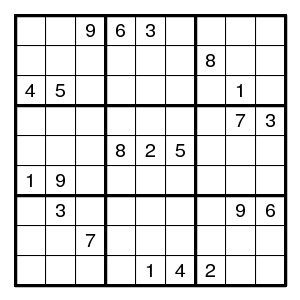By the usual arguments this is completed to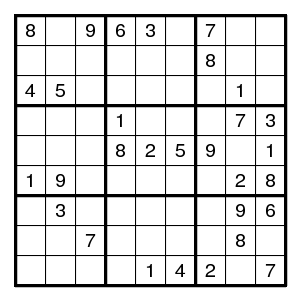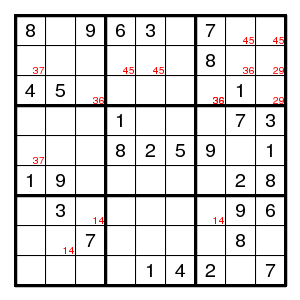In the bottom left box, the 2 must be in column 1, and we find a double pair 2,8 in the middle left box.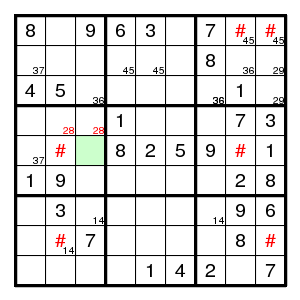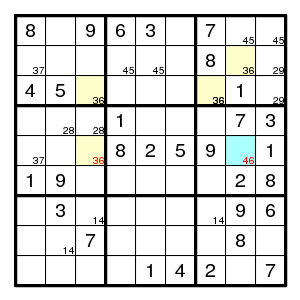The positions in columns 2,8,9 that could have a 4 have been marked. They are all on rows 1,5,8, and hence no 4 can occur on rows 1,5,8 outside columns 2,8,9. (This argument is known as swordfish.) In particular, there is no 4 at the green square. But then the alternating chain at the yellow squares shows that the blue square cannot be 6, and hence is 4. The rest is straightforward.

## Joining colours

This example was given by simes to illustrate the idea of joining colours.

Make the colouring here for the digit 4.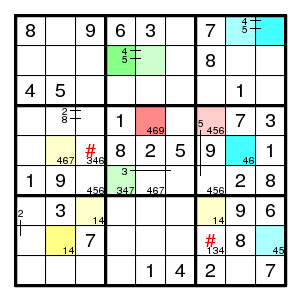There are nine connected components, five (not coloured here) consist of a single vertex only, and four are shown in yellow, blue, green and red. No immediate conclusion is obtained, but the colours yellow and blue can be joined. Indeed, on row 5 we see that lighter yellow and darker blue exclude each other, and on row 8 we see that darker yellow and lighter blue exclude each other, so that lighter yellow is true if and only if lighter blue is true. After recolouring all the blue cells yellow, we see that the two cells marked with '#' cannot have 4. (This is precisely what we concluded earlier using a swordfish.)

Of course this argument is just a special case of the use of chains of alternating pairs: (5,2)=(8,2)-(7,3)=(7,7)-(8,9)=(1,9)-(1,8)=(5,8) is such a chain, and a common neighbour of its endpoints, like (5,3), cannot have 4. One sees that alternating chains allow one to walk an odd number of steps in one colour and then cross to another.